>>

Login or Register   Integers - Arithmetic Operations
 New User Register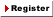Login Member: Email: Password:
 Site Search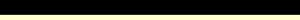Advance Search

Other animationsIntegers - Introduction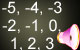Animation product List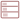Row formatGrid format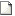List format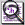Overview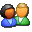(For age - group : 11 - 16 )

Fully animated ‘Integers - Arithmetic operations ’ covers the concept using real life examples. This will help you to establish rules for addition, subtraction, multiplication, division and simplification of integers. All the techniques are explained in a very detailed manner.Product - Animation
 Size/Duration Subscribe Preview / Trailer Size (KB) 5748 US\$    1.50 Rs.118.50  Check price in your currency Subscription Days = 30 Watch a preview (opens in separate window) Duration (hr:min:sec) 0:43:0 Add To Cart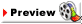This animation has audio.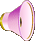Category : Math Integer Type : Animation with sound Animation Type : Regular Total animation length: 43 minutes The animation covers: Addition of integers Substraction of integers Multiplication of integers Division of integers Simplication of integers Word problems in integers Quiz section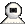Snapshots
 Watch combined previewWatch various movie clips related to integers. These are 1) Absolute value of an integer 2) Arithmetic operations of Integers 3) BODMAS Rule 4) Introduction to Integers 5) Number line - Representing History 6) Introduction to Natural and Whole Numbers 7) Representing Integers on Number line 8) Quiz on Integers. Click on the above thumbnails to go to the movie.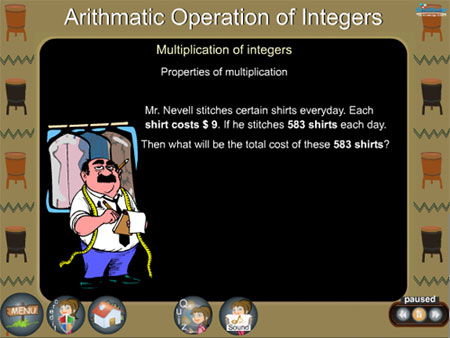The Arithmetic operation of integers covers all the mathematical operations - Addition, subtraction, division, multiplication and simplification of integers.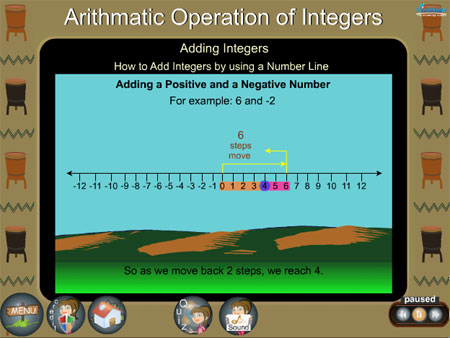This is like an animated math tutorial but with entertainment and stories to make learning integers a fun. We have provided many examples to clarify doubts in integers.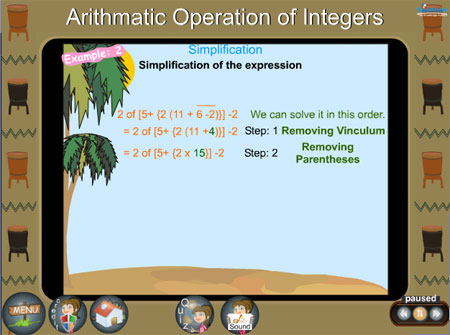The simplification of integers requires BODMAS, a technique for solving complicated expressions of integers.Details of the animation/ movie /software

Solving aritmetic operation of integers with real life problems is the basis of the animation.

Integers can be solved through a number line, where addition and subtraction operation can be plotted on a number line and the results obtained.

Addition of integers follows: Closure property, Commutative law, Associative law, Additive identity and Additive inverse. For example, when integers have different signs, the sum will have the sign of the integer with the largest absolute value.

(+8) +( -4) = +4
(-8) + (+4) = -4

When subtracting integers, change the sign on the integer that is to be subtracted or the additive inverse can be used. The additive inverse of +7 is -7 (-7 + 7 = 0).

(+7) - (-4) = (+7) + (+4) = +11
(+7) - (+4) = (+7) + (-4) = +3

Multiplication is the repeated addition of integers. If the signs are the same, the product of integers will be positive, if they are different, the product of integers will be negative.

3 x 5 = 3+3+3+3+3 = 15
3 x 5 = 15
-3 x 5 = -15
-3 x -5 = 15

In division, if the signs are the same the quotient of integers will be positive, if they are different, the quotient of integers will be negative.

4 ÷ 2 = 2
4 ÷ -2 = -2

The simplification of integers section involves the use of BODMAS

4 + 8 x 12 -25 = 4 + 96 -25 = 100 - 25 = 75

There is a quiz on word problems in integers with detailed solutions that will give a thorough understanding of the concept.

The animation covers the arithmetic operations of solving integers using real life examples; a quiz section with detailed solution also checks your understanding.Related Products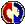Buyers Feedback:Give your comments